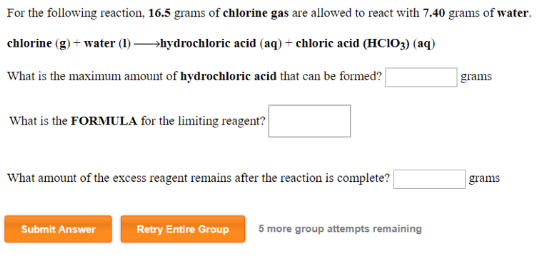# Problem: For the following reaction, 16.5 grams of chlorine gas are allowed to react with 7.40 grams of water. chlorine (g) + water (l) → hydrochloric acid (aq) + chloric acid (HCIO3) (aq) What is the maximum amount of hydrochloric acid that can be formed? What is the FORMULA for the limiting reagent? What amount of the excess reagent remains after the reaction is complete?

###### FREE Expert Solution
94% (41 ratings)###### Problem Details

For the following reaction, 16.5 grams of chlorine gas are allowed to react with 7.40 grams of water.

chlorine (g) + water (l) → hydrochloric acid (aq) + chloric acid (HCIO3) (aq)

What is the maximum amount of hydrochloric acid that can be formed?

What is the FORMULA for the limiting reagent?

What amount of the excess reagent remains after the reaction is complete?